Оценка 4.9

Оценка 4.9
doc
08.05.2020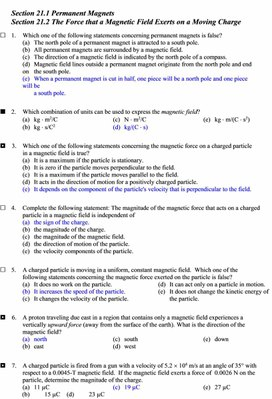Section 21.1 Permanent Magnets

Section 21.2 The Force that a Magnetic Field Exerts on a Moving Charge

Ј    1.     Which one of the following statements concerning permanent magnets is false?

(a)   The north pole of a permanent magnet is attracted to a south pole.

(b)   All permanent magnets are surrounded by a magnetic field.

(c)   The direction of a magnetic field is indicated by the north pole of a compass.

(d)   Magnetic field lines outside a permanent magnet originate from the north pole and end on        the south pole.

(e)   When a permanent magnet is cut in half, one piece will be a north pole and one piece will be

a south pole.

ў    2.     Which combination of units can be used to express the magnetic field?

(a)   kg Ч m2/C                                       (c)   N Ч m2/C                                        (e)   kg Ч m/(C Ч s2)

(b)   kg Ч s/C2                                         (d)   kg/(C Ч s)

¦    3.    Which one of the following statements concerning the magnetic force on a charged particle in a magnetic field is true?

(a)   It is a maximum if the particle is stationary.

(b)   It is zero if the particle moves perpendicular to the field.

(c)   It is a maximum if the particle moves parallel to the field.

(d)   It acts in the direction of motion for a positively charged particle.

(e)   It depends on the component of the particle's velocity that is perpendicular to the field.

Ј    4.     Complete the following statement: The magnitude of the magnetic force that acts on a charged particle in a magnetic field is independent of

(a)   the sign of the charge.

(b)   the magnitude of the charge.

(c)   the magnitude of the magnetic field.

(d)   the direction of motion of the particle.

(e)   the velocity components of the particle.

Ј    5.     A charged particle is moving in a uniform, constant magnetic field.  Which one of the following statements concerning the magnetic force exerted on the particle is false?

(a)   It does no work on the particle.                                 (d)   It can act only on a particle in motion.

(b)   It increases the speed of the particle.                       (e)   It does not change the kinetic energy of

(c)   It changes the velocity of the particle.                             the particle.

¦    6.    A proton traveling due east in a region that contains only a magnetic field experiences a vertically upward force (away from the surface of the earth). What is the direction of the magnetic field?

(a)   north                                              (c)   south                                              (e)   down

(b)   east                                                 (d)   west

¦    7.    A charged particle is fired from a gun with a velocity of 5.2 ґ 104 m/s at an angle of 35° with respect to a 0.0045-T magnetic field.  If the magnetic field exerts a force of  0.0026 N on the particle, determine the magnitude of the charge.

(a)   11 µC                                             (c)   19 µC                                             (e)   27 µC

(b)   15 µC                                             (d)   23 µC

¦    8.    Which one of the following statements best explains why a constant magnetic field can do no work on a moving charged particle?

(a)   The magnetic field is conservative.

(b)   The magnetic force is a velocity dependent force.

(c)   The magnetic field is a vector and work is a scalar quantity.

(d)   The magnetic force is always perpendicular to the velocity of the particle.

(e)   The electric field associated with the particle cancels the effect of the magnetic field on the

particle.

¦    9.    An electron traveling due south enters a region that contains a uniform magnetic field that points due east.  In which direction will the electron be deflected?

(a)   east                                                 (c)   up                                                   (e)   south

(b)   west                                                (d)   down

ў    10.  Two electrons are located in a region of space where the magnetic field is zero.  Electron A is at rest; and electron B is moving westward with a constant velocity.  A non-zero magnetic field directed eastward is then applied to the region.  In what direction, if any, will each electron be moving after the field is applied?

electron A                                   electron B

(a)   at rest                                             westward

(b)   northward                                     eastward

(c)   at rest                                             eastward

(d)   southward                                     downward, toward the earth

(e)   upward, away from earth           westward

¦    11.  An electron is moving with a speed of 3.5 ґ 105 m/s when it encounters a magnetic field of 0.60 T.  The direction of the magnetic field makes an angle of 60.0° with respect to the velocity of the electron.  What is the magnitude of the magnetic force on the electron?

(a)   4.9 ґ 10–13 N                                (c)   1.7 ґ 10–13 N                                (e)   2.9 ґ 10–14 N

(b)   3.2 ґ 10–13 N                                (d)   3.4 ґ 10–14 N

Section 21.3 The Motion of a Charged Particle in a Magnetic Field

Section 21.4 The Mass Spectrometer

¦    12.  A proton traveling due north enters a region that contains both a magnetic field and an electric field.

The electric field lines point due west.  It is observed that the proton continues to travel in a straight line due north.  In which direction must the magnetic field lines point?

(a)   up                                                   (c)   east                                                 (e)   south

(b)   down                                              (d)   west

ў    13.  An electron travels through a region of space with no acceleration.  Which one of the following statements is the best conclusion?

(a)   Both E and B must be zero in that region.

(b)   E must be zero, but B might be non-zero in that region.

(c)   E and B might both be non-zero, but they must be mutually perpendicular.

(d)   B must be zero, but E might be non-zero in that region.

(e)   E and B might both be non-zero, but they must point in opposite directions.

¦    14.  A proton is traveling south as it enters a region that contains a magnetic field.  The proton is deflected downward toward the earth.  What is the direction of the magnetic field?

(a)   downward,  toward the earth     (c)   north                                              (e)   south

(b)   west                                                (d)   east

ў    15.  A particle with a mass of 6.64 ґ 10–27 kg and a charge of +3.20 ґ 10–19 C is accelerated from rest through a potential difference of 2.45 ґ 106 V.  The particle then enters a uniform 1.60-T magnetic field.  If the particle’s velocity is perpendicular to the magnetic field at all times, what is the magnitude of the magnetic force exerted on the particle?

(a)   zero newtons                                (c)   6.55 ґ 10–10 N                              (e)   7.87 ґ 10–12 N

(b)   1.14 ґ 10–10 N                              (d)   4.09 ґ 10–11 N

 ¦    16.  An electron traveling horizontally enters a region where a uniform magnetic field is directed into the plane of the paper as shown.  Which one of the following phrases most accurately describes the motion of the electron once it has entered the field?         (a)   upward and parabolic                     (b)   upward and circular                         (c)   downward and circular         (d)   upward, along a straight line         (e)   downward and parabolic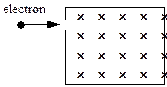ў    17.  An electron enters a region that contains a magnetic field directed into the page as shown.  The velocity vector of the electron makes an angle of 30° with the +y axis.  What is the direction of the magnetic force on the electron when it enters the field?         (a)   up, out of the page         (b)   at an angle of 30° below the positive x axis            (c)   at an angle of 30° above the positive x axis         (d)   at an angle of 60° below the positive x axis         (e)   at an angle of 60° above the positive x axis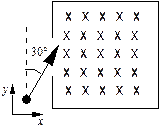¦    18.  Two particles move through a uniform magnetic field that is directed out of the plane of the page.  The figure shows the paths taken by the two particles as they move through the field.  The particles are not subject to any other forces or fields. Which one of the following statements concerning these particles is true?         (a)   The particles may both be neutral.         (b)   Particle 1 is positively charged;  2 is negative.         (c)   Particle 1 is positively charged;  2 is positive.         (d)   Particle 1 is negatively charged;  2 is negative.         (e)   Particle 1 is negatively charged;  2 is positive.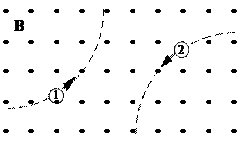ў    19.  A mass spectrometer is used to separate two isotopes of uranium with masses m1 and m2 where m2 > m1.  The two types of uranium atom exit an ion source S with the same charge of +e and are accelerated through a potential difference V.  The charged atoms then enter a constant, uniform magnetic field B as shown.  If r1 = 0.5049 m and r2 = 0.5081 m, what is the value of the ratio m1/m2?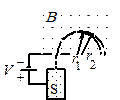(a)   0.9984                                           (c)   0.9874                                           (e)   0.9749

(b)   0.9937                                           (d)   0.9812

ў    20.  Two charged particles of equal mass are traveling in circular orbits in a region of uniform, constant magnetic field as shown.  The particles are observed to move in circular paths of radii R1 and R2 with speeds v1 and v2, respectively.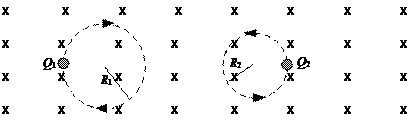As the figure shows, the path of particle 2 has a smaller radius than that of particle 1.  Which one of the following statements about this system is false?

(a)   |v1/Q1| < |v2/Q2|

(b)   Particle 2 carries a positive charge.

(c)   Particle 1 carries a negative charge.

(d)   Neither particle gains energy from the magnetic field.

(e)   The particle velocities have no components parallel to the magnetic field.

ў    21.  Two charged particles are traveling in circular orbits with the same speed in a region of uniform magnetic field that is directed into the page, as shown.  The magnitude of the charge on each particle is identical, but the signs of the charges are unequal.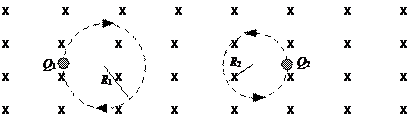Which one of the entries in the table below is correct?

 Mass Relationship Sign of charge Q1 Sign of charge Q2 (a) M1 = m2 + – (b) M1 > m2 – + (c) m1 < m2 – + (d) m1 > m2 + – (e) m1 < m2 + –

Questions 22 through 24 pertain to the situation described below:

A beam consisting of five types of ions labeled A, B, C, D, and E enters a region that contains a uniform magnetic field as shown in the figure below.  The field is perpendicular to the plane of the paper, but its precise direction is not given.  All ions in the beam travel with the same speed.  The table below gives the masses and charges of the ions.  Note: 1 mass unit = 1.67 ґ 10-27 kg and e  = 1.6 ґ 10-19 C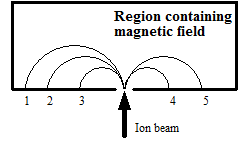Ion                  Mass               Charge   A                   2 units                  +e   B                   4 units                  +e   C                   6 units                  +e   D                   2 units                  –e   E                   4 units                  –e

ў    22.  Which ion falls at position 2?

(a)   A                                                     (c)   C                                                     (e)   E

(b)   B                                                     (d)   D

¦    23.  What is the direction of the magnetic field?

(a)   toward the right                            (c)   into the page                                 (e)   toward the bottom

(b)   toward the left                              (d)   out of the page                                     of the page

¦    24.  Determine the magnitude of the magnetic field if ion A travels in a semicircular path of radius 0.50 m at a speed of 5.0 ґ 106 m/s.

(a)   1.0 T                                               (c)   0.42 T                                            (e)   0.11 T

(b)   0.84 T                                            (d)   0.21 T

Section 21.5 The Force on a Current in a Magnetic Field

¦    25.  A 0.150-m wire oriented horizontally between the poles of an electromagnet carries a direct current of 12.5 A.  The angle between the direction of the current and that of the magnetic field is 25.0°.  If the magnetic field strength is 0.625 T, what is the magnitude and direction of the magnetic force on the wire between the poles?

 25.0°

 B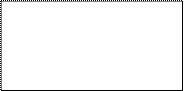I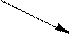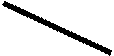(a)   1.17 N, upward                            (c)   0.792 N, upward                          (e)   0.495 N, upward

(b)   3.30 N, downward                       (d)   1.44 N, downward

¦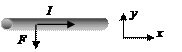26.  A long, straight wire carries a 6.0-A current that is directed in the positive x direction.  When a uniform magnetic field is applied perpendicular to a 3.0-m segment of the wire, the magnetic force on the segment is 0.36 N, directed in the negative y direction, as shown.  What are the magnitude and direction of the magnetic field?

(a)   0.020 T, out of the paper           (c)   0.060 T, out of the paper           (e)   0.65 T, out of the paper

(b)   0.020 T, into the paper               (d)   0.060 T, into the paper

 ў    27.  A loop of wire with a weight of 1.47 N is oriented vertically and carries a current I = 1.75 A.  A segment of the wire passes through a magnetic field directed into the plane of the page as shown.  The net force on the wire is measured using a balance and found to be zero.  What is the magnitude of the magnetic field? (a)   zero tesla                                       (d)   1.5 T (b)   0.51 T                                            (e)   4.2 T         (c)   0.84 T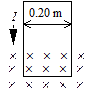Questions 28 and 29 pertain to the statement and figure below:

 A long straight vertical segment of wire traverses a magnetic field of magnitude 2.0 T in the direction shown in the diagram.  The length of the wire that lies in the magnetic field is 0.060 m.  When the switch is closed, a current of 4.0 A flows through the wire from point P to point Q.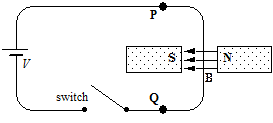¦    28.  Which one of the following statements concerning the effect of the magnetic force on the wire is true?

(a)   The wire will be pushed to the left.

(b)   The wire will be pushed to the right.

(c)   The wire will have no net force acting on it.

(d)   The wire will be pushed downward, into the plane of the paper.

(e)   The wire will be pushed upward, out of the plane of the paper.

¦    29.  What is the magnitude of the magnetic force acting on the wire?

(a)   0.12 N                                            (c)   0.48 N                                            (e)   zero newtons

(b)   0.24 N                                            (d)   67 N

Section 21.6 The Torque on a Current-Carrying Coil

Ј    30.  A current-carrying, rectangular coil of wire is placed in a magnetic field.  The magnitude of the torque on the coil is not dependent upon which one of the following quantities?

(a)   the magnitude of the current in the loop

(b)   the direction of the current in the loop

(c)   the length of the sides of the loop

(d)   the area of the loop

(e)   the orientation of the loop

¦    31.  A circular coil consists of 5 loops each of diameter 1.0 m.  The coil is placed in an external magnetic field of 0.5 T.  When the coil carries a current of 4.0 A, a torque of magnitude 3.93 N•m acts on it.  Determine the angle between the normal to the plane of the coil and the direction of the magnetic field.

(a)   0°                                                    (c)   45°                                                  (e)   90°

(b)   30°                                                  (d)   60°

 ў    32.  A single circular loop of radius 1.00 m carries a current of 10.0 mA.  It is placed in a uniform magnetic field of magnitude 0.500 T that is directed parallel to the plane of the loop as suggested in the figure.  What is the magnitude of the torque exerted on the loop by the magnetic field?         (a)   1.57 ґ 10-2 N Ч m         (b)   3.14 ґ 10-2 N Ч m         (c)   6.28 ґ 10-2 N Ч m         (d)   9.28 ґ 10-2 N Ч m         (e)   zero N Ч m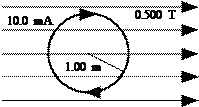ў    33.  A rectangular loop has sides of length 0.06 m and 0.08 m.  The wire carries a current of 10 A in the direction shown.  The loop is in a uniform magnetic field of magnitude 0.2 T and directed in the positive x direction.  What is the magnitude of the torque on the loop?         (a)   2 ґ 10-2 N Ч m                                      (b)   4 ґ 10-2 N Ч m                                              (c)   5 ґ 10-3 N Ч m         (d)   8 ґ 10-3 N Ч m         (e)   1 ґ 10-4 N Ч m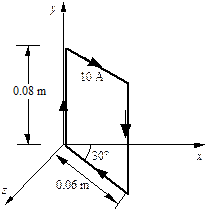¦    34.  A single circular loop of wire of radius 0.75 m carries a constant current of 3.0 A.  The loop may be rotated about an axis that passes through the center and lies in the plane of the loop.  When the orientation of the normal to the loop with respect to the direction of the magnetic field is 25°, the torque on the coil is 1.8 N Ч m.  What is the magnitude of the uniform magnetic field exerting this torque on the loop?

(a)   0.37 T                                            (c)   3.0 T                                               (e)   0.80 T

(b)   1.7 T                                               (d)   0.46 T

¦    35.  A coil consists of 240 circular loops, each of radius 0.044 m, and carries a current of 2.2 A.  Determine the magnetic moment of the coil.

(a)   0.21 A Ч m2                                   (c)   3.2 A Ч m2                                     (e)   23 A Ч m2

(b)   0.65 A Ч m2                                   (d)   15 A Ч m2

Section 21.7 Magnetic Fields Produced by Currents

Ј    36.  Which one of the following statements concerning the magnetic field inside (far from the surface) a long, current-carrying solenoid is true?

(a)   The magnetic field is zero.

(b)   The magnetic field is non-zero and nearly uniform.

(c)   The magnetic field is independent of the number of windings.

(d)   The magnetic field is independent of the current in the solenoid.

(e)   The magnetic field varies as 1/r as measured from the solenoid axis.

¦    37.  Complete the following statement: The magnetic field around a current-carrying, circular loop is most like that of

(a)   the earth.

(b)   a short bar magnet.

(c)   a current-carrying, rectangular loop.

(d)   a long straight wire that carries a current.

(e)   two long straight wires that carry currents in opposite directions.

¦    38.  A solenoid of length 0.250 m and radius 0.0200 m is comprised of 120 turns of wire.  Determine the magnitude of the magnetic field at the center of the solenoid when it carries a current of 15.0 A.

(a)   2.26 ґ 10-3 T                                (c)   9.05 ґ 10-3 T                                (e)   zero tesla

(b)   4.52 ґ 10-3 T                                (d)   7.50 ґ 10-3 T

 ў    39.  The drawing shows two long, thin wires that carry currents in the positive z direction.  Both wires are parallel to the z axis.  The 50-A wire is in the x-z plane and is 5 m from the z axis.  The 40-A wire is in the y-z plane and is 4 m from the z axis.  What is the magnitude of the magnetic field at the origin?         (a)   zero tesla                               (b)   1 ґ 10-6 T                              (c)   3 ґ 10-6 T         (d)   5 ґ 10-6 T         (e)   7 ґ 10-6 T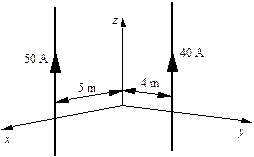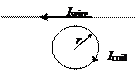ў    40.  The radius of a coil of wire with N turns is r = 0.22 m.  A clockwise current of Icoil = 2.0 A flows in the coil, as shown.  A long, straight wire carrying a current Iwire  = 31 A toward the left is located 0.05 m from the edge of the coil.  The magnetic field at the center of the coil is zero tesla.  Determine N, the number of turns.

(a)   8                                                      (c)   6                                                      (e)   1

(b)   2                                                      (d)   4

Ј    41.  A long, straight wire carries a current I.  If the magnetic field at a distance d from the wire has magnitude B, what is the magnitude of the magnetic field at a distance 2d from the wire?

(a)   B/2                                                  (c)   2B                                                   (e)   8B

(b)   B/4                                                  (d)   4B

¦    42.  An overhead electric power line carries a maximum current of 125 A.  What is the magnitude of the maximum magnetic field at a point 4.50 m directly below the power line?

(a)   5.56 ґ 10–6 T                                (c)   3.49 ґ 10–5 T                                (e)   7.95 ґ 10–3 T

(b)   1.75 ґ 10–5 T                                (d)   4.69 ґ 10–4 T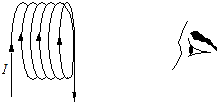Ј    43.  A coil of wire carries current I as shown in the figure.  If the observer could “see” the magnetic field inside this arrangement of loops, how would it appear?(a)                         (b)                           (c)                         (d)                         (e)

¦    44.  A wire, connected to a battery and switch, passes through the center of a long current-carrying solenoid as shown in the drawing.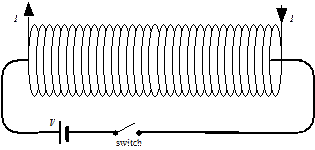When the switch is closed and there is a current in the wire, what happens to the portion of the wire that runs inside of the solenoid?

(a)   There is no effect on the wire.                           (d)   The wire is pushed into the plane of the paper.

(b)   The wire is pushed downward.                         (e)   The wire is pushed out of the plane of the paper.

(c)   The wire is pushed upward.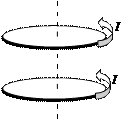ў    45.  Two loops carry equal currents I in the same direction.  The loops are held in the positions shown in the figure and are then released.  Which one of the following statements correctly describes the subsequent behavior of the loops?

(a)   Both loops move to the left.

(b)   The loops remain in the positions shown.

(c)   The top loop moves to the right; the bottom loop moves to the right.

(d)   The loops repel each other.

(e)   The loops attract each other.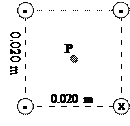ў    46.  Four long, straight wires are parallel to each other; and their cross-section forms a square.  Each side of the square is 0.020 m as shown in the figure.  If each wire carries a current of 8.0 A in the direction shown in the figure, determine the magnitude of the total magnetic field at P, the center of the square.

(a)   5.1 ґ 10-5 T                                  (d)   2.3 ґ 10-4 T

(b)   1.1 ґ 10-4 T                                  (e)   zero tesla

(c)   1.7 ґ 10-4 T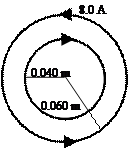¦    47.  The figure shows two concentric metal loops, each carrying a current.  The larger loop carries a current of 8.0 A and has a radius of 0.060 m.  The smaller loop has a radius of 0.040 m.  What is the value of a current in the smaller loop that will result in zero total magnetic field at the center of the system?

(a)   5.3 A                              (d)   12 A

(b)   6.0 A                              (e)   24 A

(c)   8.8 A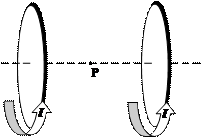¦    48.  Two loops carry equal currents I in the same direction.  They are held in the positions shown in the figure and project above and below the plane of the paper.  The point P lies exactly halfway between them on the line that joins their centers.  The centers of the loops and the point P lie in the plane of the paper.  Which one of the figures below shows the position of a compass needle if the compass were placed in the plane of the paper at P?

 S

 N

 N

(a)                                                           (c)                                                           (e)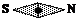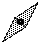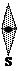S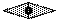N

 S

(b)                                                           (d)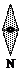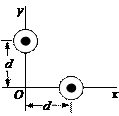ў    49.  Two long, straight wires are perpendicular to the plane of the paper as shown in the drawing.  Each wire carries a current of magnitude I.  The currents are directed out of the paper toward you.  Which one of the following expressions correctly gives the magnitude of the total magnetic field at the origin of the x, y coordinate system?

(a)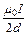(d)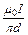(b)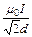(e)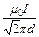(c)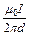Questions 50 and 51 pertain to the statement and figure below: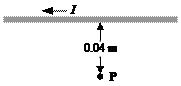A long, straight wire is carrying a current of 5.0 A in the direction shown in the figure.  The point P is 0.040 m from the wire.

Ј    50.  What is the direction of the magnetic field at point P due to the current in the wire?

(a)   to the right of page                                                      (d)   into the plane of the page

(b)   to the left of the page                                                  (e)   out of the plane of the page

(c)   toward the bottom of the page

¦    51.  What is the magnitude of the magnetic field at the point P?

(a)   1.3 ґ 10-5 T                                  (c)   2.5 ґ 10-5 T                                  (e)   9.4 ґ 10-5 T

(b)   1.9 ґ 10-5 T                                  (d)   7.9 ґ 10-5 T

Questions 52 and 53 pertain to the statement and figure below:

A single circular loop of wire with radius 0.020 m carries a current of 8.0 A.  It is placed at the center of a solenoid that has length 0.65 m, radius 0.080 m, and 1400 turns.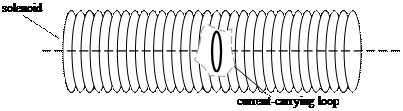ў    52.  Determine the value of the current in the solenoid so that the magnetic field at the center of the loop is zero tesla.

(a)   1.4 ґ 10-1 A                                  (c)   4.4 ґ 10-2 A                                  (e)   9.3 ґ 10-2 A

(b)   2.5 ґ 10-4 A                                  (d)   5.0 ґ 10-3 A

ў    53.  Determine the magnitude of the total magnetic field at the center of the loop (due both to the

loop and the solenoid) if the current in the loop is reversed in direction from that needed to make the total field equal to zero tesla.

(a)   2.5 ґ 10-4 T                                  (c)   6.4 ґ 10-4 T                                  (e)   9.2 ґ 10-4 T

(b)   5.0 ґ 10-4 T                                  (d)   8.7 ґ 10-4 T

Questions 54 and 55 pertain to the statement and figure below:

 Two long, straight, parallel wires separated by a distance d carry currents in opposite directions as shown in the figure.  The bottom wire carries a current of 6.0 A.  Point C is at the midpoint between the wires and point O is a distance 0.50d below the 6-A wire as suggested in the figure.  The total magnetic field at point O is zero tesla.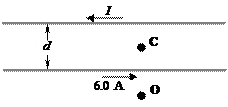ў    54.  Determine the value of the current, I, in the top wire.

(a)   2 A                                                  (c)   6 A                                      (e)   This cannot be determined since

(b)   3 A                                                  (d)   18 A                                           the value of d is not specified.

¦    55.  Determine the magnitude of the magnetic field at point C if d = 0.10 m.

(a)   2.4 Ч 10–5 T                                  (c)   9.6 Ч 10–5 T                      (e)   1.4 Ч 10–4 T

(b)   4.8 Ч 10–5 T                                  (d)   1.1 Ч 10–4 T

Questions 56 and 57 pertain to the two-wire system described below:

 Two long, straight wires separated by 0.10 m carry currents of 18 A and 6 A in the same direction as shown.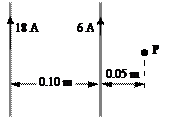¦    56.  Determine the magnitude of the magnetic field at the point P.

(a)   2.4 ґ 10–5 T                                  (c)   7.2 ґ 10–5 T                                  (e)   zero tesla

(b)   4.8 ґ 10–5 T                                  (d)   9.6 ґ 10–5 T

Ј    57.  What is the direction of the magnetic field at the point P?

(a)   to the left of the page                                                  (d)   out of the plane of the page

(b)   to the right of the page                                               (e)   into the plane of the page

(c)   toward the bottom of the page

Section 21.8 Ampere’s Law

Section 21.9 Magnetic Materials

Ј    58.  Which one of the following materials is not ferromagnetic?

(a)   iron                                                 (c)   nickel                                             (e)   cobalt

(b)   chromium dioxide                       (d)   aluminum

ў    59.  Each second, 1.25 ґ 1019 electrons in a narrow beam pass through a small hole in a wall. The beam is perpendicular to the wall.  Using Ampere’s law, determine the magnitude of the magnetic field in the wall at a radius of 0.750 m from the center of the beam.

(a)   1.18 ґ 10–9 T                                (c)   2.62 ґ 10–3 T                                (e)   3.33 ґ 1012 T

(b)   5.33 ґ 10–7 T                                (d)   4.14 ґ 108 T

Questions 60 and 61 pertain to the following:

 A long, coaxial cable, shown in cross-section in the drawing, is made using two conductors that share a common central axis, labeled C.  The conductors are separated by an electrically insulating material that is also used as the outer cover of the cable.  The current in the inner conductor is 2.0 A directed into the page and that in the outer conductor is 2.5 A directed out of the page.  The distance from point C to point A is 0.0015 m; and the distance from C to B is 0.0030 m.  The radii a and b of the conductors are 6.0 ґ 10–4 m and 1.9 ґ 10–3 m, respectively.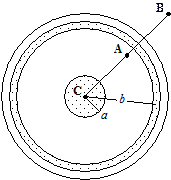ў    60.  What is the magnitude and direction of the magnetic field at point A?

(a)   3.3 ґ 10–5 T, clockwise                                               (d)   6.8 ґ 10–5 T, counterclockwise

(b)   3.3 ґ 10–5 T, counterclockwise                                 (e)   2.7 ґ 10–4 T, clockwise

(c)   6.8 ґ 10–5 T, clockwise

ў    61.  What is the magnitude and direction of the magnetic field at point B?

(a)   3.3 ґ 10–5 T, clockwise                                               (d)   6.8 ґ 10–5 T, counterclockwise

(b)   3.3 ґ 10–5 T, counterclockwise                                 (e)   2.7 ґ 10–4 T, clockwise

(c)   6.8 ґ 10–5 T, clockwise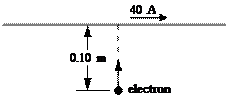Additional Problems

ў    62.  A long straight wire carries a 40.0 A current in the +x direction.  At a particular instant, an electron moving at 1.0 ґ 107 m/s in the +y direction is 0.10 m from the wire.  The charge on the electron is –1.6 ґ 10–19 C.  What is the force on the electron at this instant?

(a)   1.3 ґ 10–16 N in the +x direction                               (d)   6.5 ґ 10–10 N in the –y direction

(b)   1.3 ґ 10–16 N in the -x direction                               (e)   6.5 ґ 10–16 N in the –y direction

(c)   6.5 ґ 10–10 N in the +y direction

Questions 63 through 65 pertain to the statement and diagram below:

 A wire is bent into the shape of a circle of radius r = 0.10 m and carries a 20.0-A current in the direction shown.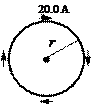Ј    63.  What is the direction of the magnetic field at the center of the loop?

(a)   to the right of the page                                               (d)   into the plane of the paper

(b)   to the left of the page                                                  (e)   out of the plane of the paper

(c)   toward the top of the page

¦    64.  What is the magnitude of the magnetic field at the center of the loop?

(a)   2.0 ґ 10–5 T                                  (c)   2.0 ґ 10–4 T                                  (e)   zero tesla

(b)   1.3 ґ 10–5 T                                  (d)   1.3 ґ 10–4 T

¦    65.  Determine the magnetic moment of the loop.

(a)   0.20 A Ч m2                                   (c)   0.63 A Ч m2                                    (e)   1.3 A Ч m2

(b)   0.40 A Ч m2                                    (d)   0.84 A Ч m2

Questions 66 and 67 pertain to the following statement and figure: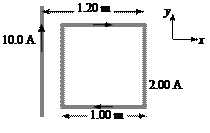A long, straight wire carries a 10.0-A current in the +y direction as shown in the figure.

Next to the wire is a square copper loop that carries a 2.00-A current as shown.  The length of each side of the square is 1.00 m.

ў    66.  What is the magnitude of the net magnetic force that acts on the loop?

(a)   8.0 ґ 10–6 N                                  (c)   1.4 ґ 10–5 N                                  (e)   2.3 ґ 10–5 N

(b)   1.1 ґ 10–5 N                                  (d)   1.7 ґ 10–5 N

ў    67.  What is the direction of the net magnetic force that acts on the loop?

(a)   +x direction                                   (c)   +y direction                                   (e)   30° with respect to

(b)   –x direction                                   (d)   –y direction                                           the +x direction

## Which one of the following statements best explains why a constant magnetic field can do no work on a moving charged particle? (a)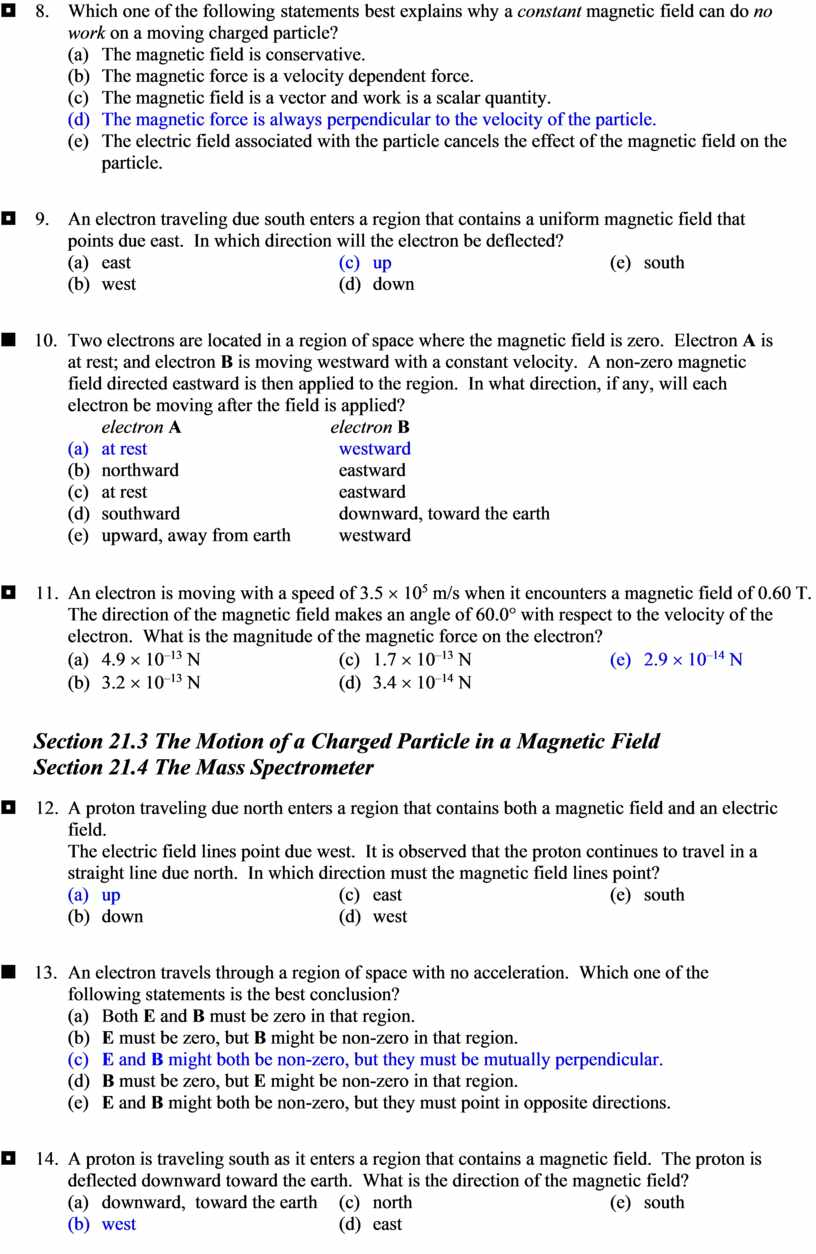## A particle with a mass of 6.64 ´ 10 –27 kg and a charge of +3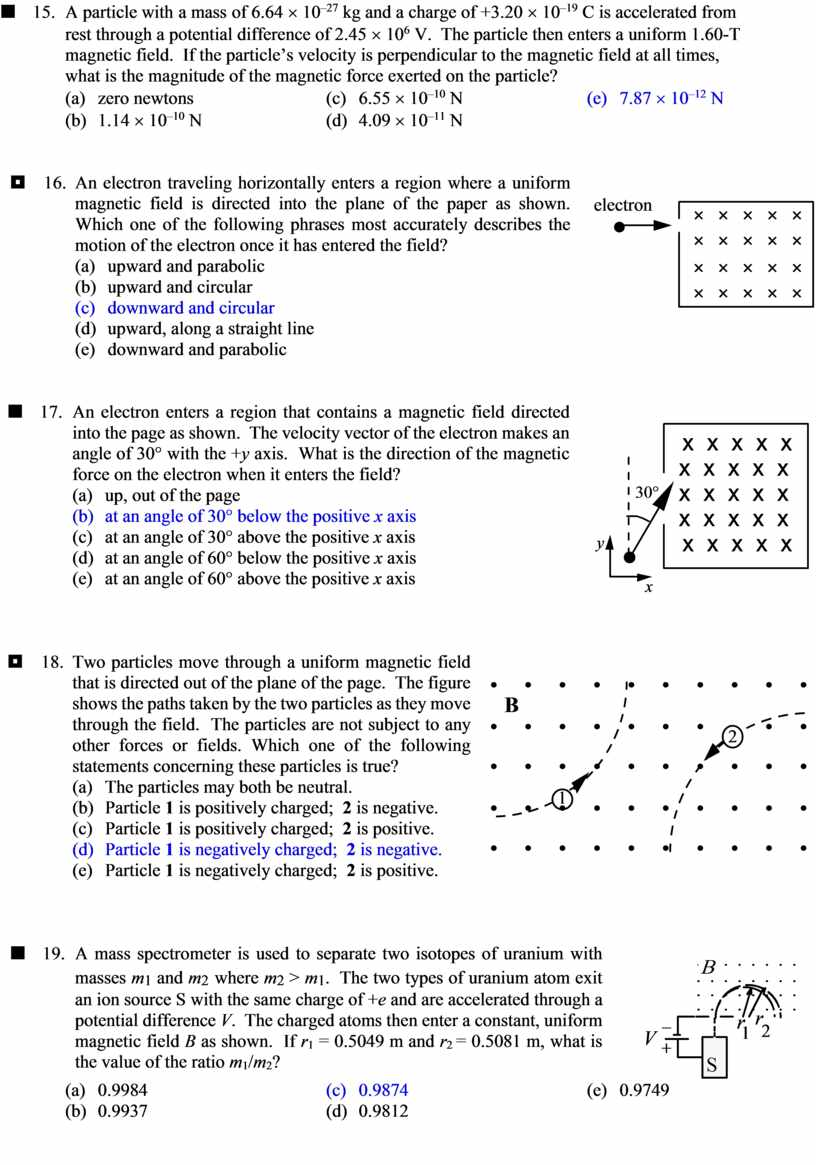## Two charged particles of equal mass are traveling in circular orbits in a region of uniform, constant magnetic field as shown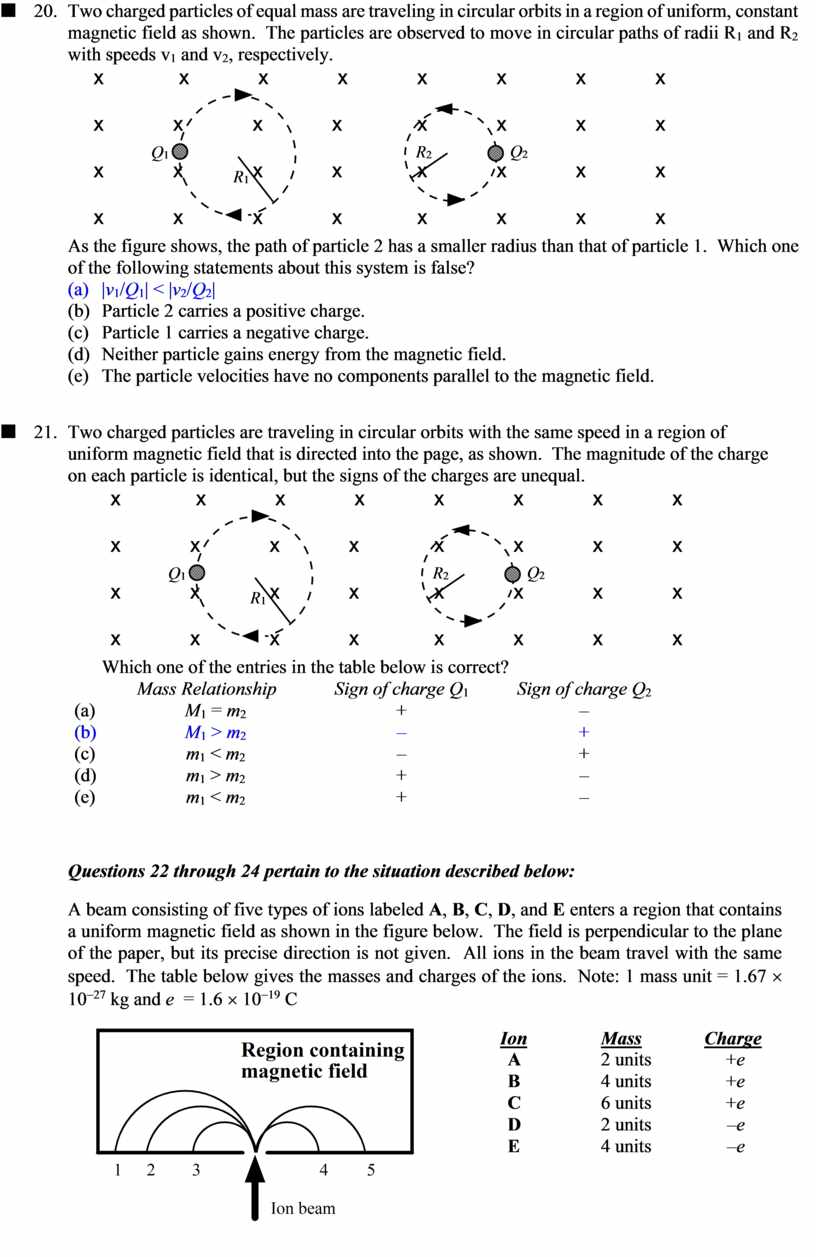## Which one of the following statements concerning the effect of the magnetic force on the wire is true? (a)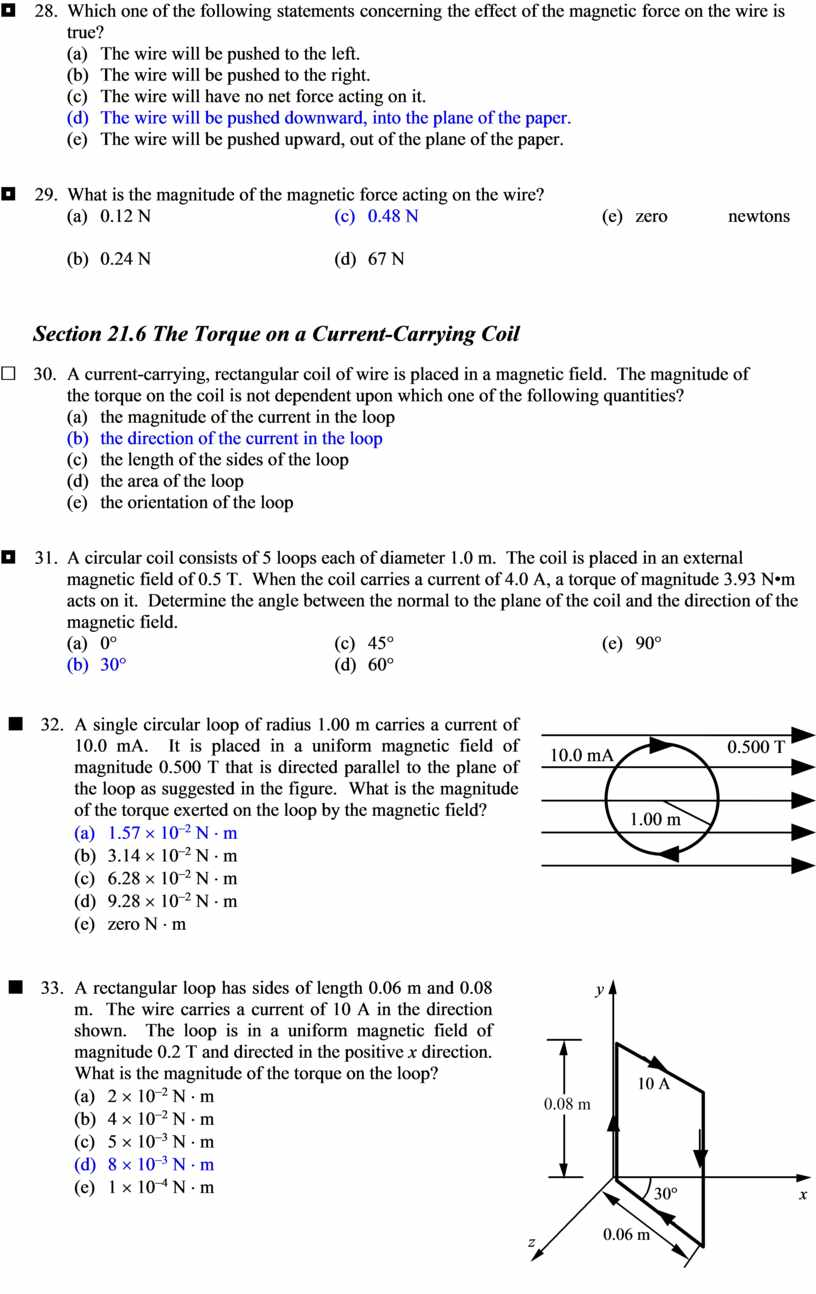## Two long, straight wires are perpendicular to the plane of the paper as shown in the drawing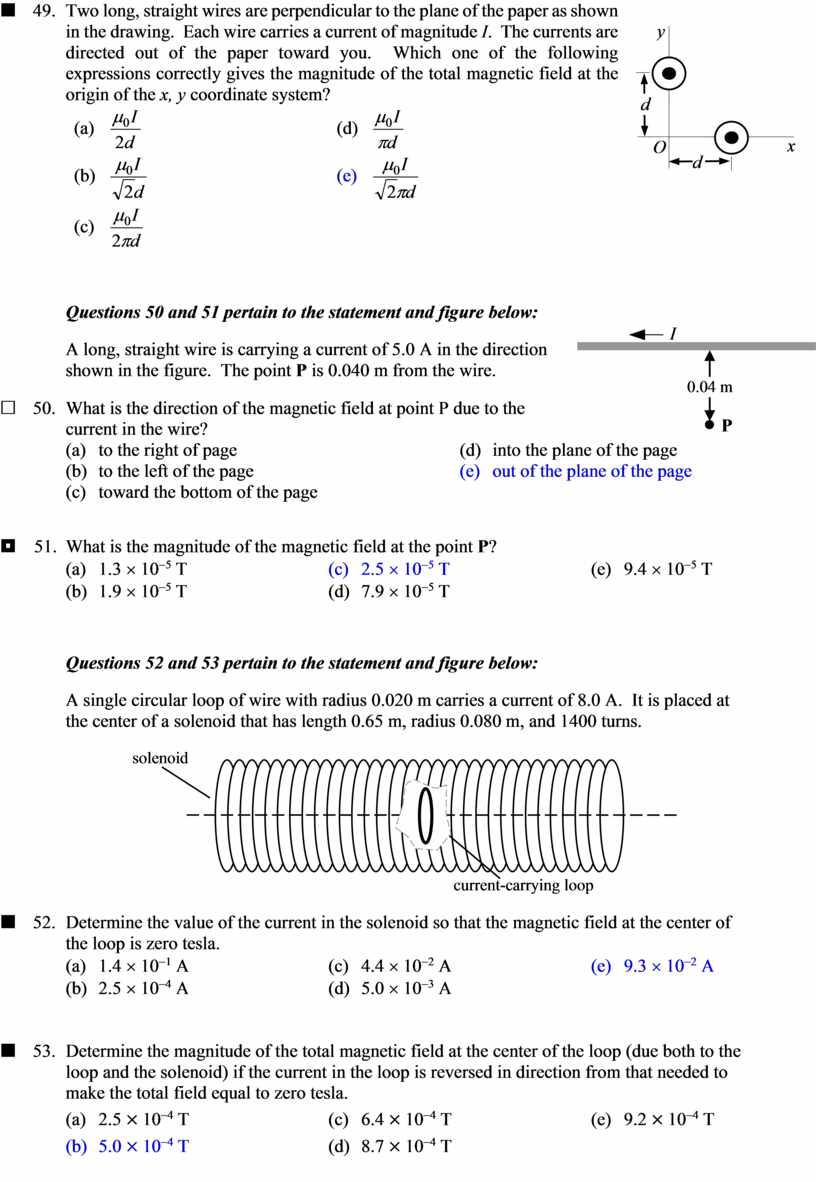## Questions 54 and 55 pertain to the statement and figure below: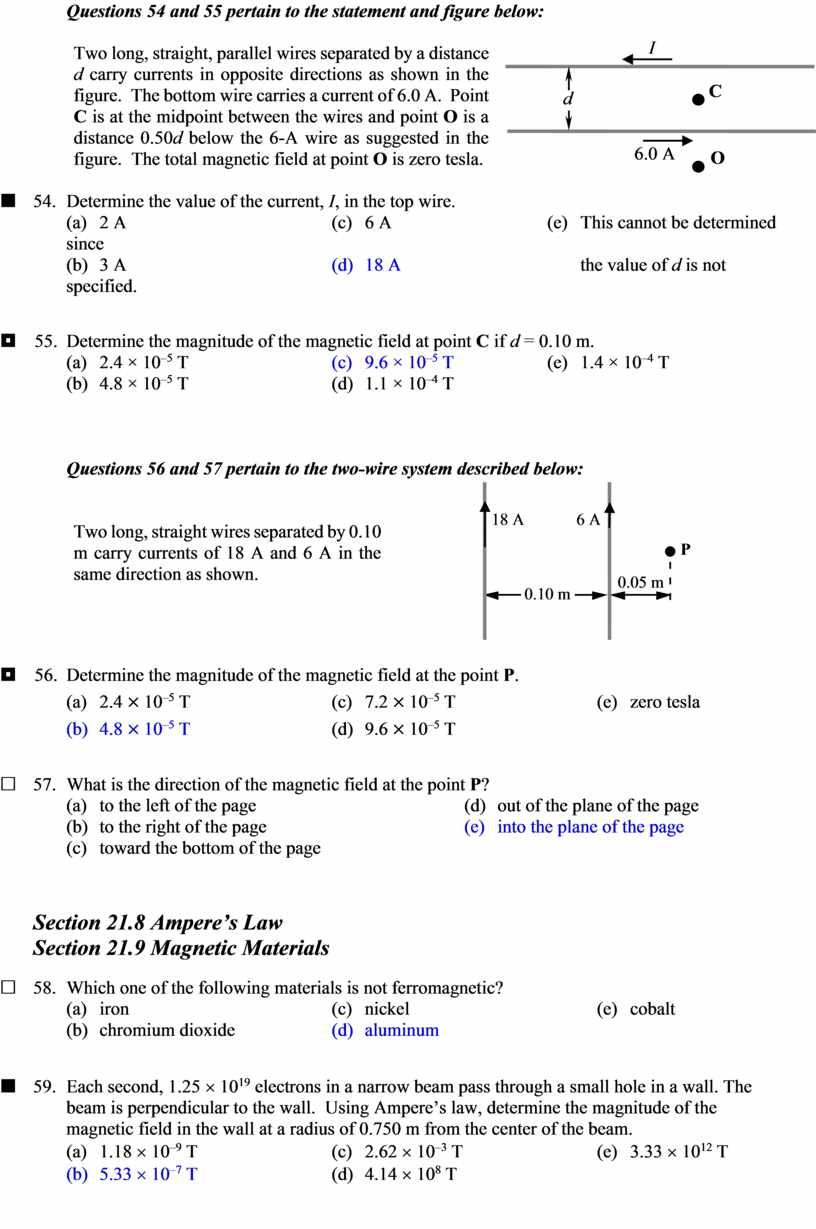Скачать файл